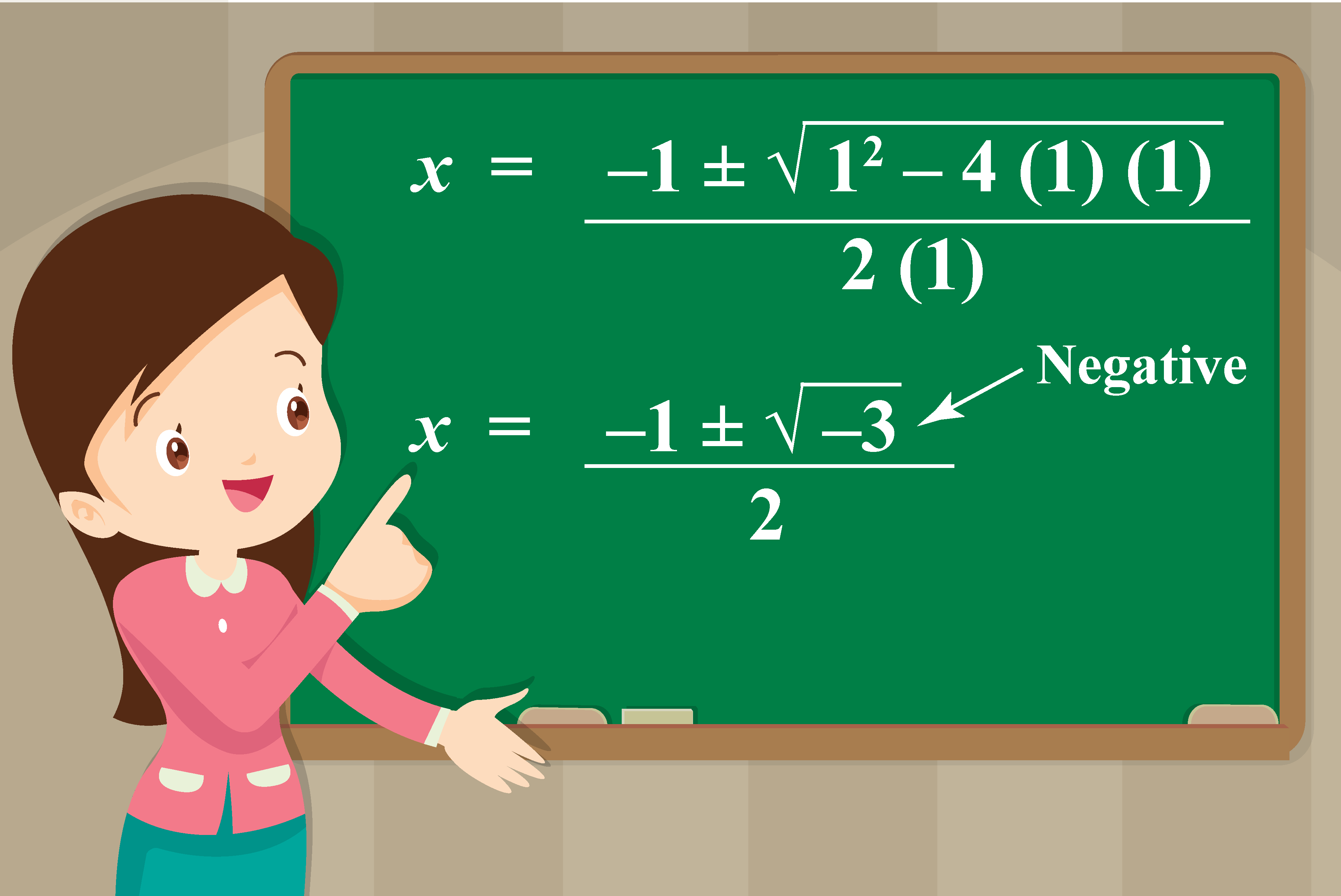# Powers of iota

 1 What is iota and Its value? 2 Square Root of iota 3 Important Notes on Powers of iota 4 Higher Powers of iota 5 Tips and Tricks on Powers of iota 6 Powers of iota Calculator 7 Solved Examples on Powers of iota 8 Practice Question 9 Maths Olympiad Sample Papers 10 Frequently Asked Questions (FAQs)

## What is Iota and Its Value?

Iota is a complex number that is denoted by $$\mathbf{i}$$ and the value of iota is $$\mathbf{\sqrt{-1}}$$.

i.e.,

 $i= \sqrt{-1}$

If we square both sides of the above equation, we get:

$i^2=-1$

i.e., the value of the square of iota is -1

While solving quadratic equations, you might have come across situations where the discriminant is negative.

For example, consider the quadratic equation

$x^2+x+1=0$

If we use the quadratic formula to solve this, we get the discriminant (the part inside the square root) as a negative value.In such cases, we write $$\sqrt{-3}$$ as:

$\sqrt{-3} =\sqrt{-1} \times \sqrt{3}= i \times \sqrt{3}= \sqrt{3}i$

This would give the solution of the above quadratic equation to be:

$x=\dfrac{-1 \pm \sqrt{3}i}{2}$

Hence, the value of iota is helpful in solving square roots with negative values.

Thus,

 The value of iota is, $$i= \sqrt{-1}$$ The square of iota is, $$i^2=-1$$

## Square Root of Iota

We will find the value of the square root of iota, i.e., $$\sqrt{i}$$

We will use De Moivre's Theorem to find this.

We know that,

\begin{align}i&= \cos \dfrac{\pi}{2}+ i \sin \dfrac{\pi}{2}\0.3cm] &= \cos \left( \dfrac{\pi}{2}+2n\pi \right)+ i \sin \left(\dfrac{\pi}{2}+2n\pi\right),n=0,1 \\[0.3cm] &= \cos \left( \dfrac{\pi+4n\pi}{2} \right)+ i \sin \left( \dfrac{\pi+4n\pi}{2} \right) \end{align} Here, we took $$n=0,1$$ as we need 2 solutions. But we have to find $$\sqrt{i}=(i)^{1/2}$$ Let us raise the exponent to $$1/2$$ on both sides. So we get: \begin{align} \sqrt{i}&=\left(\cos \left( \dfrac{\pi+4n\pi}{2} \right)+ i \sin \left( \dfrac{\pi+4n\pi}{2} \right) \right)^{1/2}\\[0.3cm] &=\cos \left( \dfrac{\pi+4n\pi}{4} \right)+ i \sin \left( \dfrac{\pi+4n\pi}{4} \right),n=0,1 \end{align} When $$n=0$$, $$\sqrt{i}= \cos \dfrac{\pi}{4}+ i \sin \dfrac{\pi}{4} = \dfrac{\sqrt{2}}{2}+ i \dfrac{\sqrt{2}}{2}$$. When $$n=1$$, $$\sqrt{i}= \cos \dfrac{5\pi}{4}+ i \sin \dfrac{5\pi}{4} = -\dfrac{\sqrt{2}}{2}- i \dfrac{\sqrt{2}}{2}$$  \[ \begin{align} \sqrt{i} &= \dfrac{\sqrt{2}}{2}+i \dfrac{\sqrt{2}}{2} \\[0.2cm] &=-\dfrac{\sqrt{2}}{2}-i \dfrac{\sqrt{2}}{2} \end{align}

## Powers of Iota Calculator

Here is the "Powers of Iota Calculator".

Here, you can enter any exponent (positive or negative) of $$i$$ and see the result in a step-by-step manner.

## Solved Examples

 Example 1

Find the values of the following:

$i^{37}, \,\,i^{99}, \,\,i^{-1}, \,\,i^{-50}$

Solution:

Since $${i^2} = - 1$$, we have:

\begin{align}&{i^3} = {i^2} \times i = - 1 \times i = - i\\&{i^4} = {\left( {{i^2}} \right)^2} = {\left( { - 1} \right)^2} = 1\end{align}

Now, we can calculate i raised to any integer power. For example,

\begin{align}&{i^{37}} = {i^{36}} \times i = {\left( {{i^4}} \right)^9} \times i = 1 \times i = i\\&{i^{99}} = {i^{96}} \times {i^3} = {\left( {{i^4}} \right)^{24}} \times {i^3} = 1 \times - i = - i\\&{i^{ - 1}} = \frac{1}{i} = \frac{i}{{{i^2}}} = \frac{i}{{ - 1}} = - i\\&{i^{ - 50}} = \frac{1}{{{i^{50}}}} = \frac{1}{{{i^{48}} \times {i^2}}} = \frac{1}{{{i^2}}} = - 1\end{align}

Thus,

 \begin{align}{i^{37}} &= i\\{i^{99}}& = - i\\{i^{ - 1}}&= - i\\{i^{ - 50}} &= - 1\end{align}
 Example 2

Find the value of $${i^{4n + k}}$$, where n and k are integers, and k is in the set {0, 1, 2, 3}

Solution:

We have:

${i^{4n + k}} = {i^{4n}} \times {i^k} = {\left( {{i^4}} \right)^n} \times {i^k} = 1 \times {i^k} = {i^k}$

Thus, the value of $${i^{4n + k}}$$ is the same as the value of $${i^k}$$, which depends on the value of k:

\begin{align}&k = 0:\; {i^k} = \;1\\&k = 1:\; {i^k} = \;i\\&k = 2:\; {i^k} = - 1\\&k = 3: \;{i^k} = - i\end{align}

Thus,

 $\begin{array}{l} k=0: i^{4n+k}=1 \\ k=1: i^{4n+k}=i \\ k=2: i^{4n+k}=-1 \\ k=3: i^{4n+k}=-i \end{array}$
 Example 3

(a) Find the value of \begin{align}{i^{500}} + {i^{501}} + {i^{502}} + {i^{503}}\end{align}

(b) Show that the sum of any four consecutive powers of iota is 0

Solution:

(a) We have:

\begin{align}&{i^{500}} = {\left( {{i^4}} \right)^{125}} = {1^{125}} = 1\\&{i^{501}} = {i^{500}} \times i = i\\&{i^{502}} = {i^{500}} \times {i^2} = {i^2} = - 1\\&{i^{503}} = {i^{500}} \times {i^3} = {i^3} = - i\end{align}

Clearly, the sum of these four terms is 0

(b) We have:

\begin{align}&{i^n} + {i^{n + 1}} + {i^{n + 2}} + {i^{n + 3}}\\&= {i^n}\left( {1 + i + {i^2} + {i^3}} \right)\\&= {i^n}\left( {1 + i - 1 - i} \right) = 0\end{align}

Thus,

## 2. What is iota cube?

$$i^3= i^2 \cdot i = (-1) \cdot i= -i$$

Thus, iota cube is $$-i$$

## 3. What is the value of i cube?

$$i^3= i^2 \cdot i = (-1) \cdot i= -i$$

Thus, $$i$$ cube is $$-i$$

Complex Numbers
grade 10 | Questions Set 1
Complex Numbers
Complex Numbers